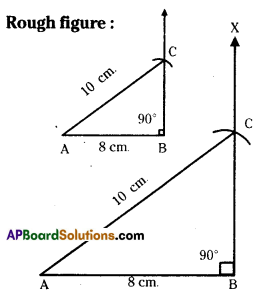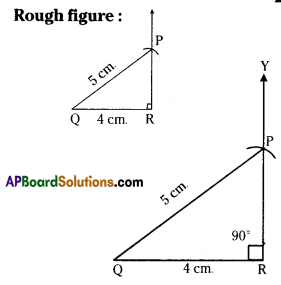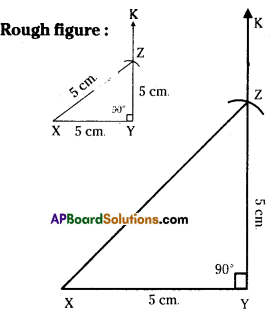# AP Board 7th Class Maths Solutions Chapter 9 Construction of Triangles Ex 4

AP State Syllabus AP Board 7th Class Maths Solutions Chapter 9 Construction of Triangles Ex 4 Textbook Questions and Answers.

## AP State Syllabus 7th Class Maths Solutions 9th Lesson Construction of Triangles Exercise 4Question 1.
Construct a right-angled ΔABC such that ∠B = 90°, AB = 8 cm and AC = 10 cm.
Solution:
∠B = 90°, AB = 8cm, AC = 10 cm.Step -1: Draw a rough sketch of the right angled triangle and label it with the given measurements.
Step -2: Draw a line segment AB of length 8 cm.
Step -3: Draw a ray $$\overrightarrow{\mathrm{BX}}$$ perpendicular to AB to B.
Step -4 : Draw an arc from A with radius 10 cm to intersect $$\overrightarrow{\mathrm{BX}}$$ at C.
Step -5: Join A, C to get the required ΔABC.Question 2.
Construct a ΔPQR, right-angled at R, hypotenuse is 5 cm and one of its adjacent sides is 4cm.
Solution:
∠R=90D,QR=4cm,PQ=5cm.Step -1: Draw a rough sketch of the right angled triangle and label it with the given measurements.
Step -2: Draw a line segment QR of length 4cm.
Step -3: Draw a ray $$\overrightarrow{\mathrm{RY}}$$ perpendicular to QR at R.
Step -4: Draw an arc from Q with radius 5 cm to intersect $$\overrightarrow{\mathrm{RY}}$$ at P.
Step -5: Join P, Q to get the required ΔPQR.Question 3.
Construct an isosceles right-angled ΔXYZ in which ∠Y = 90° and the two sides are 5 cm each.
Solution:
∠Y = 90°,XY = YZ = 5cm.Step- 1: Draw a rough sketch of a right angled triangle and label it with given measurements.
Step -2: Draw a line segment XY of length 5cm.
Step -3: Draw a ray $$\overrightarrow{\mathrm{YK}}$$ perpendicular to XY at Y.
Step -4: Draw an arc from Y with radius 5 cm to intersect $$\overrightarrow{\mathrm{YK}}$$ at Z.
Step -5: Join Z, X to get the required ΔXYZ.